# Cryptography and Reference Sequence Based DNA/RNA Sequence Compression Algorithms

Cryptography and Reference Sequence Based DNA/RNA Sequence Compression Algorithms

Siva Phanindra DaggubatiVenkata Rao Kasukurthi Prasad Reddy PVGD

Department of CS & SE, AUCE(A), Andhra University, Visakhapatnam 530003, Andhra Pradesh, India

Corresponding Author Email:
daggubatisivaphanindra@gmail.com
Page:
509-514
|
DOI:
https://doi.org/10.18280/isi.270319
1 April 2022
|
Accepted:
6 June 2022
|
Published:
30 June 2022
| Citation

OPEN ACCESS

Abstract:

This paper proposes two methods for the compression of biological sequences like DNA/RNA. Although many algorithms both lossy and lossless exist in the literature, they vary by the compression ratio. Moreover, existing algorithms show different compression ratios for different inputs. Our proposed methods exhibit nearly constant compression ratio which helps us to know the amount of storage needed in advance. For the first method, we call it CryptoCompress, we use a blend of Cryptographic hash function and partition theory to achieve this compression. The second method, we call it RefCompress, uses a reference DNA for compression. This paper showcases that the proposed methods have constant compression ratio compared to most of the existing methods.

Keywords:

CryptoCompress, RefCompress, DNA compression, reference DNA, cryptographic hash function

1. Introduction

The field of Genetics has become a branch of extensive research these days. Owing to its application in different fields, especially medical field, this has the scope of becoming a multimillion-dollar industry. This resulted in its success of attracting huge funding from various government and private agencies. The data produced in this research is growing at an exponential rate. High-throughput sequencing techniques like Pyrosequencing, cPAS, BGI/MGI, SOLID sequencing, Nanopore sequencing etc. which parallelize the computation are producing millions of sequences at low cost. This led to the demand for availability of huge storage capacity.

Although the cost of storage is decreasing, optimum usage of storage is always expected. For this, a lot of effort was put forward to compress the biological data that is pouring in at an exponential rate. Algorithms like Gen compress, Bio compress, Cfact etc. are published to compress biological data. However, their compression ratio is not constant. It varies with the input. Our proposed methods exhibit a nearly constant compression ratio. Constant compression ratio helps us in calculating the storage required in advance.

For the first method, CryptoCompress, we use two concepts. One is Cryptographic hash function and the other is Partition theory. Cryptographic hash function which is used in encrypting a message has the property that irrespective of size of the input, the size of the output is always constant. We use this property to achieve a constant compression ratio. MD5 is the function we use in this proposed technique. We use MD5because, it is easily breakable than the other functions like SHA. Partition theory that we see in number theory is used in decompression phase. Partition of a number is the number of ways a number can be expressed as the sum of other numbers. For example,

Consider the number ‘6’.6=1+5

=2+4

=1+2+3

=3+3

=1+1+1+1+1+1

=1+1+4

.............

.............

We get ‘11’partitions like this. However, we are interested in only partitions with distinct parts. In this case, we get only ‘3’partitions. This process of partitioning can be used to reconstruct the original sequence.

For the second method, RefCompress, we use a Reference DNA. This method is suitable for effective compression of human DNA. It can also be used to compress DNA of other species which have, like humans, low intra-species DNA variability.

2. Literature Review

Behzadi and Le Fessant  proposed DNAPack which can detect a better set of repeats than DNAC. Instead of greedy method that is used in DNAC, DNACPack uses dynamic programming to detect better repeats.

Beck and Alderton  describes a strategy for generating and DNA sequencing Templates.

Chen et al.  proposed DNAC algorithm. This is an improvement over Cfact algorithm. This algorithm works in four phases. First phase will construct a suffix tree, all exact repeats are extended into approximate repeats. Third phases extract optimal non-overlapping repeats and the fourth phase encodes the repeats.

Chen et al.  proposed GenCompress algorithm which achieves better compression than Biocompress and Cfact by using the measure of “relatedness” to construct evolutionary trees and follows the framework of Lempel-ziv. Hutchison  discusses about sequencing methods. Dale and Schantz  lists some of the applications of DNA technology. Loewenstern & Yianilos  presented CDNA, a statistical based compression algorithm which dependsupon probability distribution of each symbol.

Edwards et al.  discussess Matrix-assisted laser desorption ionization time-of-flight mass spectrometry (MALDI-TOF MS). Rivals et al.  proposed Cfact algorithm which constructs a suffix tree to find the longest matching exact repeat. This is a two pass version of BioCompress2 algorithm. Ziv  proposed two algorithms to compress anydata sequences. These are dictionary based compression algorithms which rely on exact repeats. Kaipa et al.  proposes an algorithm for DN A sequence compression based on Mismatch bases and repeat location. Misra et al.  proposes a DNA compression method based on horizontal and vertical compression. Franca et al.  reviews some of the sequencing techniques. Benchmark data used in this paper can be retrieved from ref. .

Rivest  proposed MD5, a Cryptographic hash function producing 128-bit hash value. Although it is intended to be used in compression, due to its vulnerabilities, it is regarded as unsafe to be used as a security tool. However, its breakable property helps us in using it in the proposed algorithm of this paper.

Grumbach and Tahi [16, 17] proposed two lossless compression algorithms Biocompress and Biocompress2 which uses the idea of Ziv . They use complimentary and exact repeats in the compression process. BioCompress2 improves BioCompress by using Arithmetic encoding to improve the compression. Srinivasa et al.  proposes a dynamic programming approach for D NA compression. Human Genome can be downloaded from ref. .

In this paper, we propose two algorithms Cryptocompress and RefCompress which achieves, unlike the other algorithms, a constant compression ratio irrespective of the input apart from achieving better compression in some of the cases. We compare Cryptocompress with Cfact, GenCompress and GenCompress2 algorithms. Regarding RefCompress, it is a proposal which was not implemented. However, its theoretical concept gives us the confidence to believe in its proposed ability.

3. Tools and Resources

The aim of this paper is to propose Biological sequence, especially DNA sequence compression algorithms that can achieve constant compression ratio irrespective of the size of the input. This constant compression ratio helps us in predicting the amount of space needed to store the sequences. The method we adopted is Experimental in nature. We used standard benchmark sequences used in ref.  to apply for CryptoCompress, one of the proposed algorithms. The MD5 algorithm, an essential part in first method comes as a handy tool for our approach. Its vulnerability of being easily broken comes as a boon for our method. The compression ratio that we use to compare the methods is similar to the one defined in ref.  ie1-(|O|/2|I|), where ‘O’ is the number of bits in output sequence and ‘I’ is the size of the input. Although many of the proposed compression methods are influenced by Limpel-Ziv algorithms, we get our idea from Cryptography and Partition theory. We use the data in ref.  to compare our algorithm with Cfact, GenComporess, GenCompress2 and other algorithms. For the second proposed algorithm, RefCompress, we use Human Reference Genome published in ref. . This algorithm describes the method of compressing Human DNA sequences by comparing the input sequence with the reference sequence. Since it is difficult to get real data about Human DNA, RefCompress is a theoretical proposal which is not implemented.

The rest of the paper is organized as follows. ‘4’ gives the algorithm of CryptoCompress, the first proposed method. ‘5’ explains RefCompress, the second method. In \6\, we analyze the results of applying CryptoCompress to the data in ref. . Conclusion gives a brief description of what we have done in this paper.

4. CryptoCompress Algorithm

4.1 Compression

First, the input sequence which has a four letter alphabet (A, G, T, C) is subjected to trivial substitution as follows.

A=10 G=01 T=00 C=11.

Then, it is divided into blocks of 256 bits. For explanation, we shall take a small sequence. Let’s consider the sequence ATTCTTAG. It can be written as 1000001100001001.Let us call it encrypted sequence E.

E=1000001100001001.

Since ‘E’ contains only 16-bits, we consider this as a block. (However, practically we will consider up to 256 bits as a block). Now for each block of 256 bits, do the following:

Add the positions of 1’s in ‘É’. We get the sum:

S=1+7+8+13+16=45.

This sum ‘45’ can be represented in 15-bit binary as 000000000101101. We use 15 bits because a 256 bit sequence can produce a maximum sum of 32896 which can be expressed with 15 bits.

Now apply MD5 hash function on ‘É’. We get:

MD5 (E)=8ea04cb4f49277939ceebc7e551b54f5.

We get 128-bit hash value. For this we augment the sum ‘S’.

The final compressed block is:

C1=MD5(E) ||S= 8ea04cb4f49277939ceebc7e551b54f545.

This ‘C’ is stored as 143-bit (128+15) binary string. After completing this process for every block, the final compressed string can be made by augmenting all the compressed blocks.

Compressed string C=C1||C2||C3||....Cn.

Thus each block of 256 bits is compressed to 143 bits. 256 bits binary string represents 1024-bit original sequence. Thus the original sequence is compressed by 86.3%.

The Compression is summarized as follows (Figure 1).

## 1.png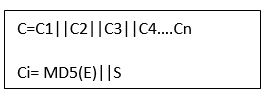Figure 1. Compression stage in CryptoCompress

4.1.1 Compression algorithm

Input: DNA/RNA sequence.

Output: Compressed sequence.

Step1: Trivial step. Let A=00, G=01, T=10, C=11. Replace the bases in the input sequence with the corresponding binary encoding. Call this Encoded sequence “E”.

Step 2: Initialize the compressed sequence “C” to null.

Step 3: Consider 256 bits of Encoded sequence “E” as a block. Divide “E” into blocks.

Step 4: For each block, Iterate step 5 and step 6.

Step 5: Calculate the message digest of the block using MD5 algorithm.

Step 6: Add the positions of 1’s in the input block and append the resulting sum (15 bit) to the message digest. The resultant 143(128+15) bit sequence is appended to the compressed sequence “C”.

Step 7: The resultant “C” is the compressed sequence.

4.2 Decompression

In decompression phase, we divide the compressed sequence into blocks of 143 bits. Now for each block, do the following.

Consider the last 15-bits as sum ‘S’.

In the given example:

S=45= 000000000101101 in binary.

Now find all the partitions of 45 with distinct parts. For each partition, construct the corresponding binary sequence by placing 1’s in the positions of parts of the partition and 0’s in other places.

For Ex, 45=1+44.So corresponding binary sequence is M=1000000000000000000000000000000000000000000010000upto 256bits.

Apply MD5 cryptographic hash function on the generated sequence ieMD5 (M). This is compared with the first 128-bits. If they both are equal, we consider ‘M’ as the sequence ‘É1’ produced after trivial encryption of a block of the original sequence. In this example, we get the equality for the partition.

45= 1+7+8+13+16.

The corresponding binary sequence is:

E1=1000001100001001

After applying the above process for every block, we construct the original trivially encoded sequence by augmenting the result of all blocks.

E=E1||E2||E3||E4....

Now the original sequence can be retrieved by replacing bits as follows.

10=A 01=G 00=T 11=C.

In our example, if we apply this for ‘E’, we get ATTCTTAG which is the original sequence.

4.2.1 Decompression algorithm

Input: Compressed sequence “C”.

Output: Original Sequence.

Step 1: Consider the size of a block as 143 bits. Divide the input into blocks.

Step 2: initialize the Encoded sequence “E” to null.

Step 3: Generate the next block. If no block can be generated, go to step 10.

Step 4: Consider the last 15 bits as the sum “S” of positions of 1’s in the encoded sequence “E”.

Step 5: Find the number of partitions of ’S’ with distinct parts that uses integers from 1 to 256 only.

Step 6: Generate the next partition.

Step 7: Generate the binary sequence for the partition.

Step 8: Apply MD5 algorithm for this sequence and compare it with the remaining 128 bits in the block.

Step 9: If the two matches, append the binary sequence to the Encoded sequence “E” and goto step 3,

Else goto step6

Step 10: Let 00=A, 01=G, 10=T, 11=C. Substitute the corresponding characters in the Encoded sequence.

“E”

Step 11: The resultant sequence is the decompressed original sequence.

5. RefCompress Algorithm

This method is highly useful in compressing DNA of human like species which have low intra species DNA variability. As per the data available, any two humans differ in their chromosomal DNA by almost 0.6%. We use this property to compress the sequence. Our human DNA is 3 billion bases long. To store it we need 3GB space. To compress it, we can use Human reference DNA sequence readily available in public databases like NCBI.

5.1 Compression

We shall explain the method with an example. Since we cannot take a 3 billion long sequence here, we will explain the method by taking the Reference sequence and the uncompressed sequence as 10 bases long.

Reference Sequence: GTCCTAGCTA

Uncompressed Sequence: GTCCGAGCTA

We have taken one base difference between the two sequences. Practically, in a ten base sequence, the difference should be less than one base. However, to facilitate the explanation, we take it to be one base.

We compare each base in uncompressed string to each base in Reference string. If both of them match, we add a “0” to output string. If they don’t match, we add a ‘1’ in the output sequence followed by “00” for the base ‘A’ in uncompressed string, “01” for ‘G’, “11” for ‘T’, “10” for ‘C’.

Applying this method for the above string, we get the output sequence as

Output Sequence: 000011000000

The original string in our example is 10bytes long. Our compressed string is 1.5 bytes long. Applying the same to Human DNA, the original DNA of 3GB will be reduced to a minimum of 388 MB, i.e., we can expect a minimum compression of 87%.

5.1.1 Compression algorithm

Input: Reference Genome Ref, DNA Sequence to be compressed Gen.

Output: Compressed Sequence Com.

For each base Gen[i],

if Gen[i]=Ref[i], then

Com +=0.

else

Com +=1

if(Gen[i]=’A’)

Com+=00

if(Gen[i]=’G’)

Com+=01

if(Gen[i]=’T’)

Com+=11

if(Gen[i]=’C’)

Com+=10

5.2 Decompression

Decompression is straight forward. The compressed sequence, which is converted to binary is analysed one bit at a time. If the bit is ‘0’, the corresponding base in the Reference DNA is substituted in the output. If it is ‘1’, the next two bits give the base to be substituted.

5.2.1 Decompression algorithm

Input: Compressed Sequence Com

Output: Original Sequence Gen

For each bit ‘i’ in Com

if(Com[i]=0)

Gen+=Ref[i]

else

if(Com[i+1]=0 and Com[i+2]=0)

Gen+=’A’

if(Com [i]=0 and Com[i+2]=1)

Gen +=’G’

if(Com [i]=1 and Com[i+2]=1)

Gen +=’T’

if(Com [i]=1 and Com[i+2==0)

Gen +=’C’

i+=2

5.2.2 Algorithm analysis

RefCompress described above is most suitable for human like creatures. It relies on the fact that humans have very low variance in their DNA. Although this method doesn’t give us a constant compression ratio, it provides us nearly constant compression due to the fact that we don’t vary much in our DNA. Since, it compares every base in the given sequence, it has a time-complexity of O(n).

6. Results

The following section discusses the results obtained by implementing CryptoCompress algorithm. Regarding RefCompress algorithm, as mentioned earlier, lack of real data constraints it to proposal. CryptoCompress is applied to standard benchmark data taken from . Results of applying algorithms like LZW, Arith2, Cfact, GenCompress1 and GenCompress2 is directly taken from . The following graphs Figure 2, Figure 3, Figure 4, Figure 5, Figure 6, Figure 7, Figure 8 and Figure 9 show the compression obtained by our CryptoCompress algorithm when compared to other algorithms listed above. In the following graphs, X-axis denotes various compression algorithms and Y-axis denotes the compression ratio expressed as percentage.

## 2.pngFigure 2. Compression ratios of atatsgs

## 3.png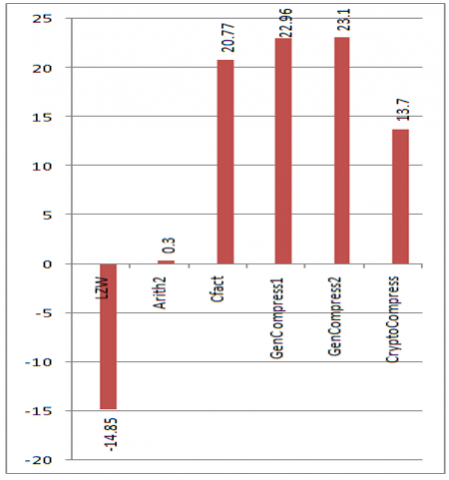Figure 3. Compression ratios of atefla23

## 4.png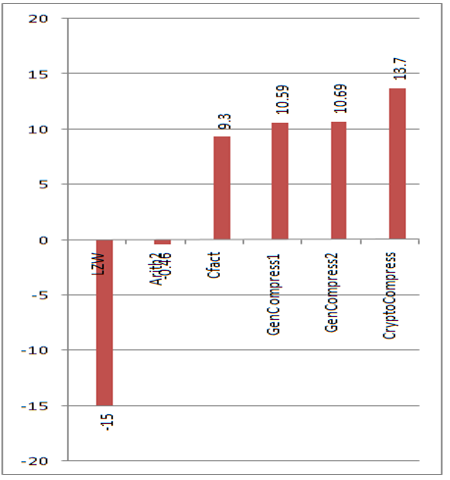Figure 4. Compression ratios of atrdnaf

## 5.pngFigure 5. Compression ratios of atrdnai

## 6.png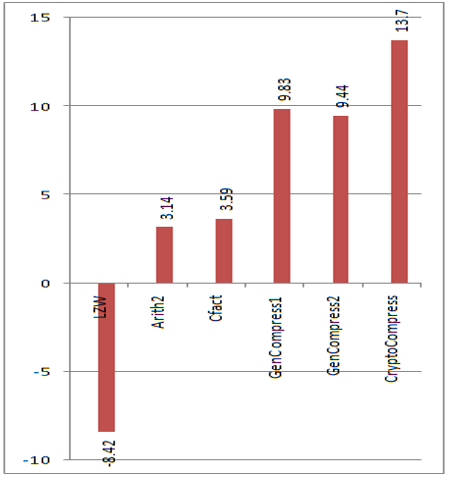Figure 6. Compression ratios of hsg6pdgen

## 7.png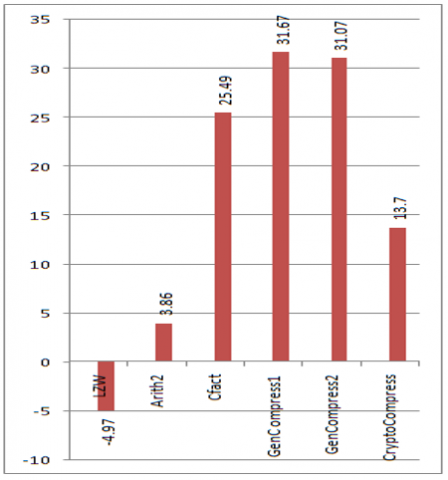Figure 7. Compression ratios of xlxfg512

## 8.png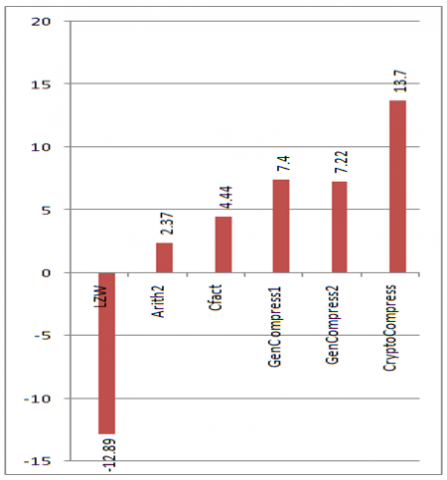Figure 8.Compression ratios of mmzp3g

## 9.png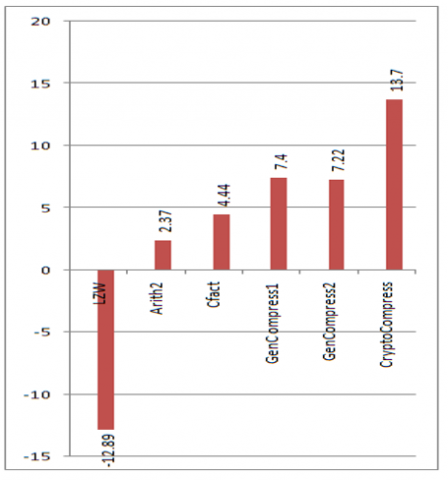Figure 9. Compression ratios of celk07e12

We can clearly observe that although our algorithm, Crypto Compress, doesn’t show better compression in all the cases, it did achieve a constant compression (86.3%). This optimizes the cost of storage as the needed storage can be easily calculated. However, the time complexity is the problem. The comparisons needed in the decompression phase cannot be predicted. This makes the algorithm compromise the time in place of space. This could be decreased by choosing the initial encryption wisely. Suppose, if the input sequence contains more ‘Á’ alphabet, it could be substituted with ‘00’. Thus by precalculating the frequency of the four bases in the input sequence, we could wisely choose the substitution so that the bases with high frequencies is replaced with more 0’s. This may decrease (not in all cases) the time taken by decompression phase. Using parallel processing to generate all the partitions would be another solution.

7. Conclusion

This paper discussed two methods, one cryptographic based (CryptoCompress) and the other based on Reference DNA (RefCompress). We established that these techniques can achieve a nearly constant compression ratio irrespective of the input sequence. We also discussed their drawback of having high time complexity. Further research can be made to reduce this time complexity of the algorithms proposed.

References

 Behzadi, B., Le Fessant, F. (2005). DNA Compression Challenge Revisited: A Dynamic Programming Approach. http://www.cs.ucr.edu/~stelo/cpm/cpm05/cpm05_5_2_Behzadi.pdf.

 Beck, S., Alderton, R.P. (1993). A strategy for amplification, purification, and selection of M13 templates for large-scale DNA sequencing. Anal Biochem., 212(2): 498-505. https://doi.org/10.1006/abio.1993.1359

 Chen, X., Li, M., Ma, B., Tromp, J. (2002). DNACompress: Fast and effective DNA sequence compression. Bioinformatics, 18(12): 1696-1698. https://doi.org/10.1093/bioinformatics/18.12.1696

 Chen, X., Kwong, S., Li, M. (1999). A compression algorithm for DNA sequences and its applications in genome comparison. Genome informatics. International Conference on Genome Informatics, 10: 51-61. http://dx.doi.org/10.1145/332306.332352

 Hutchison, C.A. (2007). DNA sequencing: bench to bedside and beyond. Nucleic Acids Res., 35(18): 6227-6237. https://doi.org/10.1093/nar/gkm688

 Dale, J.W., Schantz, M.V. (2008). From Genes to Genomes Concepts and Applications of DNA Technology. 2nd Edition, Wiley.

 Loewenstern, D., Yianilos, P.N. (1997). Significantly lower entropy estimates for natural DNA sequences. In Proc. of the Data Compression Conf., (DCC '97), pp. 151-160. https://doi.org/10.1109/DCC.1997.581998

 Edwards, J.R., Ruparel, H., Ju, J.Y. (2005). Mass-spectrometry DNA sequencing. Mutation Research/Fundamental and Molecular Mechanisms of Mutagenesis, 573(1-2): 3-12. http://dx.doi.org/10.1016/j.mrfmmm.2004.07.021

 Rivals, E., Delahaye, J.P., Dauchet, M., Delgrange, O. (1996). A guaranteed compression scheme for repetitive DNA sequences. Data Compression Conference. https://doi.ieeecomputersociety.org/10.1109/DCC.1996.488385

 Ziv, J. (1977). A universal algorithm for sequential data compression. IEEE Trans. Inform. Theory, 23(3): 337-343.

 Kaipa, K.K., Bopardikar, A.S., Abhilash, S., Venkataraman, P., Lee, K., Ahn, T., Narayanan, R. (2010). Algorithm for DNA sequence compression based on prediction of mismatch bases and repeat location. IEEE Conference on Bioinformatics and Biomedicine Workshop (BIBMW), pp. 851-852. https://doi.org/10.1109/BIBMW.2010.5703941

 Misra, K.N., Aaggarwal, A., Abdelhadi, E., Srivastava, P. (2010). An efficient horizontal and vertical method for online DNA sequence compression. International Journal of Computer Applications, 3(1). http://dx.doi.org/10.5120/757-954

 Franca, L.T.C., Carrilho, E., Kist, T.B.L. (2002). A review of DNA sequencing techniques. Quarterly Reviews of Biophysics, 35(2): 169-200. https://doi.org/10.1017/S0033583502003797

 National Center for Bio Technology Information. https://www.ncbi.nlm.nih.gov/htbin-post/Entrenz/query?db=n_s.

 Rivest, R. (1992). Step 4. Process Message in 16-Word Blocks. The MD5 Message-Digest Algorithm. https://doi.org/10.17487/RFC1321

 Grumbach, S., Tahi, F. (1994). A new challenge for compression algorithms: Genetic sequences. Journal of Information Processing and Management, 30(6): 875-866. https://doi.org/10.1016/0306-4573(94)90014-0

 Grumbach, S., Tahi, F. (1993). Compression of DNA sequences. In Proc. IEEE Symp. On Data Compression, Snowbird, UT, USA, pp. 340-350. https://doi.org/10.1109/DCC.1993.253115

 Srinivasa, K.G., Jagadish, M., Venugopal, K.R., Patnaik, L.M. (2006). Efficient compression of non repetitive DNA sequences using dynamic programming. 2006 International Conference on Advanced Computing and Communications, pp. 569-574. https://doi.org/10.1109/ADCOM.2006.4289956

 Homo sapiens (human). https://www.ncbi.nlm.nih.gov/genome/?term=human, accessed on 18 April 2022.